# Subband and Wavelet

Coding of still images under MPEG-4  and the recent decision by the JPEG committee to recommend a new standard under JPEG2000  has brought up a new image compression technique. The committee has decided to recommend a new way of coding still images based on the wavelet transform, in sharp contrast to the discrete cosine transform (DCT) used in the other standard codecs, as well as the original JPEG. In this Chapter we introduce this wavelet transform and show how it can be used for image compression.

## Why wavelet transform?

Before describing the wavelet transform and its usage in image compression it is essential to answer two fundamental questions:

1. What is wrong with the DCT and why should we use wavelet?
2. If wavelet is superior to DCT, why did the original JPEG not use it?

The answer to the first part is as follows. The DCT and the other block-based transforms partition an image into nonoverlapping blocks and process each block separately. At very low bit rates, the transform coefficients need to be coarsely quantised and so there will be a significant reconstruction error after the decoding. This error is more visible at the block boundaries, by causing a discontinuity in the image, and is best known as the blocking artefact. One way of reducing this artefact is to allow the basis functions to decay towards zero at these points, or to overlap over the adjacent blocks. The latter technique is called the lapped orthogonal transform . The wavelet transform is a special type of such transform and hence it is expected to eliminate blocking artefacts.

The answer to the second part relates to the state of the art in image coding in the mid 1980s, the time when the original JPEG was under development. At that time although the wavelet transform and its predecessor, subband coding, were known, there was no efficient method of coding the wavelet transform coefficients, to be comparable with the DCT. In fact the proposals submitted to the JPEG committee were all DCT-based codecs, none on the wavelet. Also almost at the same time, out of the 15 proposals to the H.261 committee, there were 14 DCT-based codecs and one vector quartisation method and none on the wavelet transform. Thus in the mid 1980s, the compression performance, coupled with the considerable momentum already behind the DCT, led the JPEG committee to adopt DCT-based coding as the foundation of JPEG.

However, the state of the wavelet-transform-based image compression techniques have significantly improved since the introduction of the original JPEG. Much of the credit should go to Jussef Shapiro, who by the introduction of the embedded zero tree wavelet (EZW) made a significant breakthrough in coding of the wavelet coefficients . At the end of this Chapter this method, and the other similar methods that exploit the multiresolution properties of the wavelet transform to give a computationally simple but efficient compression algorithm, will be introduced.

## Subband coding

Before describing the wavelet transform, let us look at its predecessor, subband coding, which sometimes is called the early wavelet transform . As we will see later, in terms of image coding they are similar. However, subband coding is a product designed by engineers , and the wavelet transform was introduced by mathematicians . Therefore before proceeding to mathematics, which is sometimes cumbersome to follow, an engineering view of multiresolution signal processing may help us to understand it better.

Subband coding was first introduced by Crochiere et al. in 1976 , and has since proved to be a simple and powerful technique for speech and image compression. The basic principle is the partitioning of the signal spectrum into several frequency bands, then coding and transmitting each band separately. This is particularly suited to image coding. First, natural images tend to have a nonuniform frequency spectrum, with most of the energy being concentrated in the lower frequency band. Secondly, human perception of noise tends to fall off at both high and low frequencies and this enables the designer to adjust the compression distortion according to perceptual criteria. Thirdly, since images are processed in their entirety, and not in artificial blocks, there is no block structure distortion in the coded picture, as occurs in the block-transform-based image encoders, such as DCT.

Thus subband, like the Fourier transform, is based on frequency domain analysis of the image but its filter banks have a better decorrelation property that suits natural images better. This can be explained as follows. Fourier basis functions are very exact in frequency, but are spatially not precise. In other words, the signal energy of Fourier basis functions is not concentrated at one frequency, but is spread over all space. This would not be a problem if image pixels were always correlated. However, in reality, pixels in images of interest generally have low correlation, especially across the image discontinuities such as edges. In contrast to Fourier basis functions, the subband bases not only have fairly good frequency concentration but are also spatially compact. If image edges are not too closely packed, most of the subband basis elements will not intersect with them, thus performing a better decorrelation on average.

In subband coding the band splitting is done by passing the image data through a bank of bandpass analysis filters, as shown in Figure 4.1. In order to adapt the frequency response of the decomposed pictures to the characteristics of the human visual system, filters are arranged into octave bands.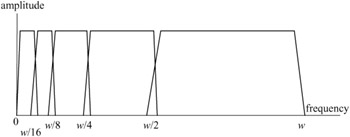Figure 4.1: A bank of bandpass filters

Since the bandwidth of each filtered version of the image is reduced, they can now in theory be downsampled at a lower rate, according to the Nyquist criteria, giving a series of reduced size subimages. The subimages are then quantised, coded and transmitted. The received subimages are restored to their original sizes and passed through a bank of synthesis filters, where they are interpolated and added to reconstruct the image.

In the absence of quantisation error, it is required that the reconstructed picture should be an exact replica of the input picture. This can only be achieved if the spatial frequency response of the analysis filters tiles the spectrum (i.e. the individual bands are set one after the other) without overlapping, which requires infinitely sharp transition regions and cannot be realised practically. Instead, the analysis filter responses have finite transition regions and do overlap, as shown in Figure 4.1, which means that the downsampling/upsampling processes introduce aliasing distortion into the reconstructed picture.

In order to eliminate the aliasing distortion, the synthesis and analysis filters have to have certain relationships such that the aliased components in the transition regions cancel each other out. To see how such a relation can make alias-free subband coding possible, consider a two-band subband, as shown in Figure 4.2.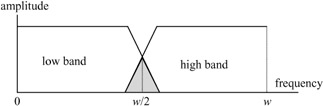Figure 4.2: A two-band analysis filter

The corresponding two-band subband encoder/decoder is shown in Figure 4.3. In this diagram, filters H0(z) and H1(z) represent the z transform transfer functions of the respective lowpass and highpass analysis filters. Filters G0(z) and G1(z) are the corresponding synthesis filters. The downsampling and upsampling factors are 2.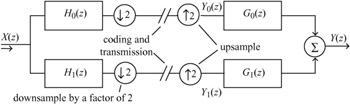Figure 4.3: A two-band subband encoder/decoder

At the encoder, downsampling by 2 is carried out by discarding alternate samples, the remainder being compressed into half the distance occupied by the original sequence. This is equivalent to compressing the source image by a factor of 2, which doubles all the frequency components present. The frequency domain effect of this downsampling/compression is thus to double the width of all components in the sampled spectrum.

At the decoder, the upsampling is a complementary procedure: it is achieved by inserting a zero-valued sample between each input sample, and is equivalent to a spatial expansion of the input sequence. In the frequency domain, the effect is as usual the reverse and all components are compressed towards zero frequency.

The problem with these operations is the impossibility of constructing ideal, sharp cut analysis filters. This is illustrated in Figure 4.4a. Spectrum A shows the original sampled signal which has been lowpass filtered so that some energy remains above Fs/4, the cutoff of the ideal filter for the task. Downsampling compresses the signal and expands to give B, and C is the picture after expansion or upsampling. As well as those at multiples of Fs, this process generates additional spectrum components at odd multiples of Fs/2. These cause aliasing when the final subband recovery takes place as at D.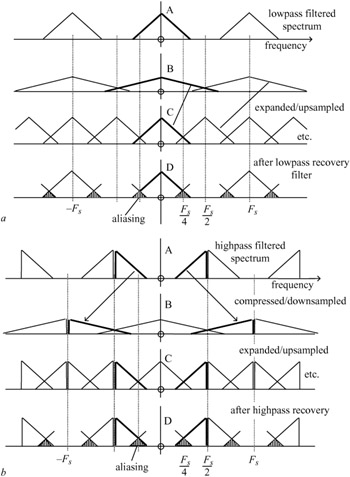Figure 4.4: (a) lowpass subband generation and recovery (b) highpass subband generation and recovery

In the highpass case, Figure 4.4b, the same phenomena occur, so that on recovery there is aliased energy in the region of Fs/4. The final output image is generated by adding the lowpass and highpass subbands regenerated by the upsamplers and associated filters. The aliased energy would normally be expected to cause interference. However, if the phases of the aliased components from the high and lowpass sub-bands can be made to differ by π, then cancellation occurs and the recovered signal is alias free.

How this can be arranged is best analysed by reference to z transforms. Referring to Figure 4.3, after the synthesis filters, the reconstructed output in z transform notation can be written as:

 (4.1)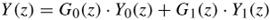where Y0(z) and Y1(z) are inputs to the synthesis filters after upsampling. Assuming there are no quantisation and transmission errors, the reconstructed samples are given by:

 (4.2)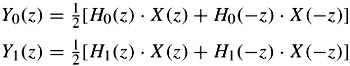where the aliasing components from the downsampling of the lower and higher bands are given by H0(-z)X(-z) and H1(-z)X (-z), respectively. By substituting these two equations in the previous one, we get:

 (4.3)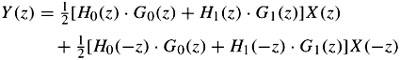The first term is the desired reconstructed signal, and the second term is aliased components. The aliased components can be eliminated regardless of the amount of overlap in the analysis filters by defining the synthesis filters as:

 (4.4)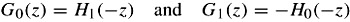With such a relation between the synthesis and analysis filters, the reconstructed signal now becomes:

 (4.5)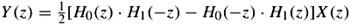If we define P(z) = H0(z)H1(-z), then the reconstructed signal can be written as:

 (4.6)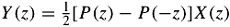Now the reconstructed signal can be a perfect, but an m-sample delayed, replica of the input signal if:

 (4.7)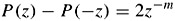Thus the z transform input/output signals are given by:

 (4.8)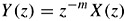This relation in the pixel domain implies that the reconstructed pixel sequence {y(n)} is an exact replica of the input sequence but delayed by m pixels, {i.e. x(n - m)}.

In these equations P(z) is called the product filter and m is the delay introduced by the filter banks. The design of analysis/synthesis filters is based on factorisation of the product filter P(z) into linear phase components H0(z) and H1(-z), with the constraint that the difference between the product filter and its image should be a simple delay. Then the product filter must have an odd number of coefficients. LeGall and Tabatabai  have used a product filter P(z) of the kind:

 (4.9)and by factorising have obtained several solutions for each pair of the analysis and synthesis filters:

 (4.10)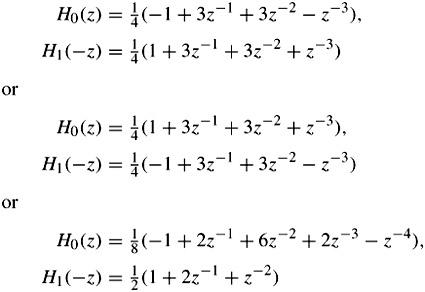The synthesis filters G0(z) and G1(z) are then derived using their relations with the analysis filters, according to eqn. 4.4. Each of the above equation pairs gives the results P(z) - P(-z) = 2z-3, which implies that the reconstruction is perfect with a delay of three samples.

## Wavelet transform

The wavelet transform is a special case of subband coding and is becoming very popular for image and video coding. Although subband coding of images is based on frequency analysis, the wavelet transform is based on approximation theory. However, for natural images that are locally smooth and can be modelled as piecewise polynomials, a properly chosen polynomial function can lead to frequency domain analysis, like that of subband. In fact, wavelets provide an efficient means for approximating such functions with a small number of basis elements. Mathematically, a wavelet transform of a square-integrable function x(t) is its decomposition into a set of basis functions, such as:

 (4.11)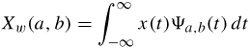where ψa,b(t) is known as the basis function, which is a time dilation and translation version of a bandpass signal Ψ(t), called the mother wavelet and is defined as:

 (4.12)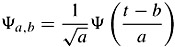where a and b are time dilation and translation parameters, respectively. The effects of these parameters are shown in Figure 4.5.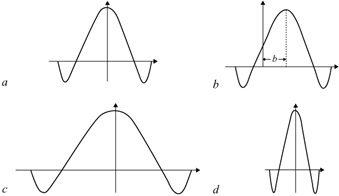Figure 4.5: Effect of time dilation and translation on the mother wavelet (a) mother wavelet Ψ(t) = Ψ1,0(t), a = 1, b = 0 (b) wavelet Ψ1,b(t), a = 1, b ≠ 0 (c) wavelet Ψ 2,0(t) at scale a = 2, b = 0 (d) wavelet Ψ 0.5,0(t) at scale a = 1/2, b = 0

The width of the basis function varies with the dilation factor (or scale) a. The larger is a, the wider becomes the basis function in the time domain and hence narrower in the frequency domain. Thus it allows varying time and frequency resolutions (with trade-off between both) in the wavelet transform. It is this property of the wavelet transform that makes it suitable for analysing signals having features of different sizes as present in natural images. For each feature size, there is a basis function Ψa,b(t) in which it will be best analysed. For example: in a picture of a house with a person looking through a window, the basis function with larger a will analyse conveniently the house as a whole. The person by the window will be best analysed at a smaller scale and the eyes of the person at even smaller scale. So the wavelet transform is analogous to the analysis of a signal in the frequency domain using bandpass filters of variable central frequency (which depends on parameter a) but with a constant quality factor. Note that in filters, the quality factor is the ratio of the centre frequency to the bandwidth of the filter.

### Discrete wavelet transform (DWT)

As the wavelet transform defined in eqn. 4.11 maps a one-dimensional signal x(t) into a two-dimensional function Xw(a, b), this increase in dimensionality makes it extremely redundant, and the original signal can be recovered from the wavelet transform computed on the discrete values of a and b . The a can be made discrete by choosing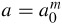, with a0 > 1 and m an integer. As a increases, the bandwidth of the basis function (or frequency resolution) decreases and hence more resolution cells are needed to cover the region. Similarly, making b discrete corresponds to sampling in time (sampling frequency depends on the bandwidth of the signal to be sampled which in turn is inversely proportional to a), it can be chosen as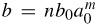. For a0 = 2 and b0 = 1 there are choices of Ψ(t) such that the function Ψm,n(t) forms an orthonormal basis of space of square integrable functions. This implies that any square integrable function x(t) can be represented as a linear combination of basis functions as:

 (4.13)where αm,n are known as the wavelet transform coefficients of x(t) and are obtained from eqn. 4.11 by:

 (4.14)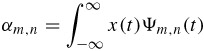It is interesting to note that for every increment in m, the value of a doubles. This implies doubling the width in the time domain and halving the width in the frequency domain. This is equivalent to signal analysis with octave band decomposition and corresponds to dyadic wavelet transform similar to that shown in Figure 4.1, used to describe the basic principles of subband coding, and hence the wavelet transform is a type of subband coding.

### Multiresolution representation

Application of the wavelet transform to image coding can be better understood with the notion of multiresolution signal analysis. Suppose there is a function Φ(t) such that the set Φ (t - n), n ∈ Z is orthonormal. Also suppose Φ(t) is the solution of a two-scale difference equation:

 (4.15)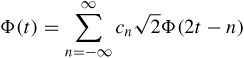where

 (4.16)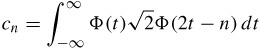Let x(t) be a square integrable function, which can be represented as a linear combination of Φ(t - n) as:

 (4.17)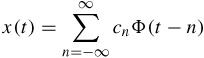where cn is the expansion coefficient and is the projection of x(t) onto Φ(t - n). Since dilation of a function varies its resolution, it is possible to represent x(t) at various resolutions by dilating and contracting the function Φ(t). Thus x(t) at any resolution m can be represented as:

 (4.18)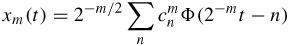If Vm is the space generated by 2-m/2Φ(2-mt - n), then from eqn. 4.15, Φ(t) is such that for any i > j, the function that generates space Vi is also in Vj, i.e. ViVj (i > j). Thus spaces at successive scales can be nested such that Vm for increasing m can be viewed as the space of decreasing resolution. Therefore, a space at a coarser resolution Vj-1 can be decomposed into two subspaces: a space at a finer resolution Vj and an orthogonal complement of Vj, represented by Wj such that Vj + Wj = Vj-1 where WjVj. The space Wj is the space of differences between the coarser and the finer scale resolutions, which can be seen as the amount of detail added when going from a smaller resolution Vj to a larger resolution Vj-1. The hierarchy of spaces is depicted in Figure 4.6.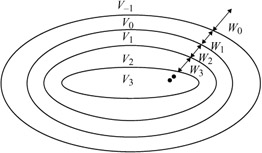Figure 4.6: Multiresolution spaces

Mallat  has shown that in general the basis for Wj consists in translations and dilations of a single prototype function Ψ (t), called a wavelet. Thus, Wm is the space generated by Ψm,n(t) = 2-m/2Ψ(2-m t - n). The wavelet Ψ(t) ∈ V_1 can be obtained from Φ(t) as:

 (4.19)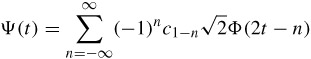The function Φ(t) is called the scaling function of the multiresolution representation. Thus the wavelet transform coefficients of eqn. 4.14 correspond to the projection of x(t) onto a detail space of resolution m, Wm. Hence, a wavelet transform basically decomposes a signal into spaces of different resolutions. In the literature, these kinds of decomposition are in general referred to as multiresolution decomposition. Here is an example of calculating the Haar wavelet through this technique.

Example: Haar wavelet

The scaling function of the Haar wavelet is the well known rectangular (rect) function: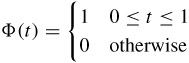The rect function satisfies eqn. 4.15 with cn calculated from eqn. 4.16 as:Thus using eqn. 4.19, the Haar wavelet can be found as: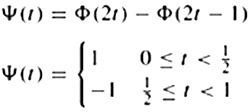The scaling function Φ(t) (a rect function) and the corresponding Haar wavelet Ψ(t) for this example are shown in Figure 4.7a and b, respectively. In terms of the approximation perspective, the multiresolution decomposition can be explained as follows. Let x(t) be approximated at resolution j by function Ajx(t) through the expansion series of the orthogonal basis functions. Since Wj represents the space of difference between a coarser scale Vj_1 and a finer scale Vj, then Djx(t) ∈ Wj represents the difference of approximation of x(t) at the j - 1th and jth resolution (i.e. Djx(t) = Aj-1x(t) - Ajx(t)).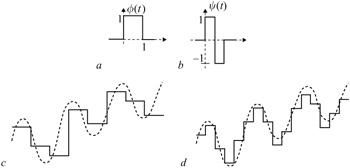Figure 4.7: (a) Haar scaling function (b) Haar wavelet (c) approximation of a continuous function, x(t), at coarser resolution A0x(t) (d) higher resolution approximation A1x(t)

Thus the signal x(t) can be split as x(t) = A-1x(t) = A0x(t) + D0x(t).

Figure 4.7c and d show the approximations of a continuous function at the two successive resolutions using a rectangular scaling function. The coarser approximation A0x(t) is shown in Figure 4.7c and at higher resolution approximation A1x(t) in Figure 4.7d where the scaling function is a dilated version of the rectangular function. For a smooth function x(t), most of the variation (signal energy) is contained in A0x(t), and D0x(t) is nearly zero. By repeating this splitting procedure and partitioning A0x(t) = A1x(t) + D1x(t), the wavelet transform of signal x(t) can

be obtained and hence the original function x(t) can be represented in terms of its wavelets as:

 (4.20)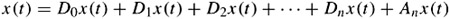where n represents the number of decompositions. Since the dynamic ranges of the detail signals Djx(t) are much smaller than the original function x(t), they are easier to code than the coefficients in the series expansion of eqn. 4.13.

### Wavelet transform and filter banks

For the repeated splitting procedure described above to be practical, there should be an efficient algorithm for obtaining Djx(t) from the original expansion coefficient of x(t). One of the consequences of multiresolution space partitioning is that the scaling function Φ(t) possesses a self similarity property. If Φ(t) and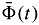are the analysis and synthesis scaling functions, and Ψ(t) and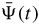are analysis and synthesis wavelets, then since VjVj-1, these functions can be recursively defined as:

 (4.21)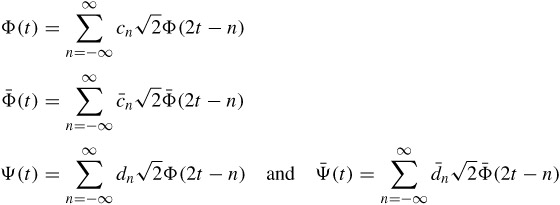These recurrence relations provide ways of calculating the coefficients of the approximation of x(t) at resolution j, Ajx(t), and the coefficients of the detail signal Djx(t) from the coefficients of the approximation of x(t) at a higher resolution Aj-1x(t). In fact, simple mathematical manipulations can reveal that both the coefficients of approximation at a finer resolution and detail coefficients can be obtained by convolving the coefficient of approximation at a coarser resolution with a filter and downsampling it by a factor of 2. For a lower resolution approximation coefficient, the filter is a lowpass filter with taps hk = c_k, and for the details the filter is a highpass filter with taps gk = d_k. Inversely, the signal at a higher resolution can be recovered from its approximation at a lower resolution and coefficients of the corresponding detail signal. It can be accomplished by upsampling the coefficients of approximation at a lower resolution and detail coefficients by a factor of 2, convolving them with the synthesis filters of taps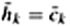and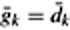, respectively, and adding them together. One step of splitting and inverse process is shown in Figure 4.8, which is in fact the same as Figure 4.3 for subband. Thus the filtering process splits the signals into lowpass and highpass frequency components and hence increases frequency resolution by a factor of 2, but downsampling reduces the temporal resolution by the same factor. Hence, at each successive step, better frequency resolution at the expense of temporal resolution is achieved.Figure 4.8: One stage wavelet transform (a) analysis (b) synthesis

### Higher order systems

The multidimensional wavelet transform can be obtained by extending the concept of the two-band filter structure of Figure 4.8 in each dimension. For example, decomposition of a two-dimensional image can be performed by carrying out one-dimensional decomposition in the horizontal and then in the vertical directions.

A seven-band wavelet transform coding of this type is illustrated in Figure 4.9, where band splitting is carried out alternately in the horizontal and vertical directions. In the Figure, L and H represent the lowpass and highpass analysis filters with a 2:1 downsampling, respectively. At the first stage of dyadic decomposition, three subimages with high frequency contents are generated. The subimage LH1 has mainly low horizontal, but high vertical frequency image details. This is reversed in the HL1 subimage. The HH1 subimage has high horizontal and high vertical image details. These image details at a lower frequency are represented by the LH2, HL2 and HH2 bands, respectively. The LL2 band is a lowpass subsampled image, which is a replica of the original image, but at a smaller size.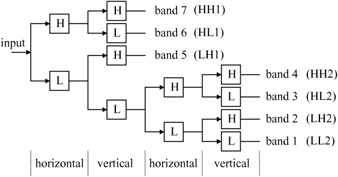Figure 4.9: Multiband wavelet transform coding using repeated two-band splits

### Wavelet filter design

As we saw in section 4.3.3, in practice the wavelet transform can be realised by a set of filter banks, similar to those of the subband. Relations between the analysis and synthesis of the scaling and wavelet functions also follow those of the synthesis and analysis filters of the subband. Hence we can use the concept of the product filter, defined in eqn. 4.7, to design wavelet filters. However, if we use the product filter as was used for the subband, we do not achieve anything new. But, if we add some constraints on the product filter, such that the property of the wavelet transform is maintained, then a set of wavelet filters can be designed.

One of the constraints required to be imposed on the product filter P(z) is that the resultant filters H0(z) and H1(z) be continuous, as required by the wavelet definition. Moreover, it is sometimes desirable to have wavelets with the largest possible number of continuous derivatives. This property in terms of the z transform means that the wavelet filters and consequently the product filter should have zeros at z = -1. A measure of the number of derivatives or number of zeros at z = -1 is given by the regularity of the wavelets and also called the number of vanishing moments . This means that in order to have regular filters, filters must have a sufficient number of zeros at z = -1, the larger the number of zeros, the more regular the filter is.

Also since, in images, phase carries important information it is necessary that filters must have linear phase responses. On the other hand, although the orthogonal filters have an energy preserving property, most of the orthogonal filters do not have phase linearity. A particular class of filters that have linear phase in both their analysis and synthesis and are very close to orthogonal are known as biorthogonal filters. In biorthogonal filters, the lowpass analysis and the highpass synthesis filters are orthogonal to each other and similarly the highpass analysis and the lowpass synthesis are orthogonal to each other, hence the name biorthogonal. Note that, in the biorthogonal filters, since the lowpass and the highpass of either analysis or synthesis filters can be of different lengths, they are not themselves orthogonal to each other.

Thus for a wavelet filter to have at least n zeros at z = -1, we chose the product filter to be :

 (4.22)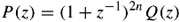where Q(z) has n unknown coefficients. Depending on the choice of Q(z) and the regularity of the desired wavelets, one can design a set of wavelets as desired. For example with:

n = 2 and Q(z) = -1 + 4z-1 - z-2

the product filter becomes:

 (4.23)and with a proper weighting for orthonormality and then factorisation, it leads to two sets of (5, 3) and (4, 4) filter banks of eqn. 4.10. The weighting factor is determined from eqn. 4.7. These filters were originally derived for subbands, but as we see they can also be used for wavelets. Filter pair (5, 3) is the recommended filter for the lossless image coding in JPEG2000 . The coefficients of its analysis filters are given in Table 4.1.

Table 4.1: Lowpass and highpass analysis filters of integer (5, 3) biorthogonal filter

n

lowpass

highpass

0

6/8

+1

±1

2/8

-1/2

±2

-1/8

As another example with:

 (4.24)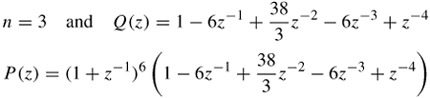the (9,3) pair of Daubechies filters  can be derived, which are given in Table 4.2. These filter banks are recommended for still image coding in MPEG-4 .

Table 4.2: Lowpass and highpass analysis filters of Daubechies (9, 3) biorthogonal filter

n

lowpass

highpass

0

0.99436891104360

0.70710678118655

±1

0.41984465132952

-0.35355339059327

±2

-0.17677669529665

±3

-0.06629126073624

±4

0.03314563036812

Another popular biorthogonal filter is the Duabechies (9,7) filter bank, recommended for lossy image coding in the JPEG2000 standard . The coefficients of its lowpass and highpass analysis filters are tabulated in Table 4.3. These filters are known to have the highest compression efficiency.

Table 4.3: Lowpass and highpass analysis filters of Daubechies (9, 7) biorthogonal filter

n

lowpass

highpass

0

0.85269865321930

-0.7884848720618

±1

0.37740268810913

0.41809244072573

±2

-0.11062402748951

0.04068975261660

±3

-0.02384929751586

-0.06453905013246

±4

0.03782879857992

The corresponding lowpass and highpass synthesis filters can be derived from the above analysis filters, using the relationship between the synthesis and analysis filters given by eqn. 4.4. That is G0(z) = H1(-z) and G1(z) = -H0(-z).

Example

As an example of the wavelet transform, Figure 4.10 shows all the seven subimages generated by the encoder of Figure 4.9 for a single frame of the flower garden test sequence, with a nine-tap low and three-tap highpass analysis Daubechies filter pair, (9, 3), given in Table 4.2. These filters have been recommended for coding of still images in MPEG-4 , which has been shown to achieve good compression efficiency.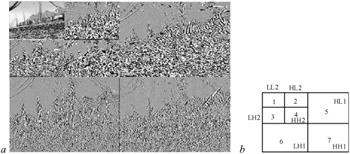Figure 4.10: (a) the seven subimages generated by the encoder of Figure 4.9 (b) layout of individual bands

The original image (not shown) dimensions were 352 pixels by 240 lines. Bands 1–4, at two levels of subdivision, are 88 by 60, and bands 5–7 are 176 by 120 pixels. All bands but band 1 (LL2) have been amplified by a factor of four and an offset of +128 to enhance visibility of the low level details they contain. The scope for bandwidth compression arises mainly from the low energy levels that appear in the highpass subimages.

Since at image borders all the input pixels are not available, a symmetric extension of the input texture is performed before applying the wavelet transform at each level . The type of symmetric extension can vary. For example in MPEG-4, to satisfy the perfect reconstruction conditions with the Daubechies (9, 3) tap analysis filter pairs, two types of symmetric extension are used.

Type A is only used at the synthesis stage. It is used at the trailing edge of lowpass filtering and the leading edge of highpass filtering stages. If the pixels at the boundary of the objects are represented by abcde, then the type A extension becomes edcba|abcde, where the letters in bold type are the original pixels and those in plain are the extended pixels. Note that for a (9,3) analysis filter pair of Table 4.2, the synthesis filter pair will be (3, 9) with G0(z) = H1(-z) and G1(z) = -H0(-z), as was shown in eqn. 4.4.

Type B extension is used for both leading and trailing edges of the low and highpass analysis filters. For the synthesis filters, it is used at the leading edge of the lowpass, but at the trailing edge of the highpass. With this type of extension, the extended pixels at the leading and trailing edges become edcb|abcde and abcde|dcba, respectively.

## Coding of the wavelet subimages

The lowest band of the wavelet subimages is a replica of the original image, but at a much reduced size, as can be seen from Figure 4.10. Efficient coding of this band depends on the number of wavelet decomposition levels. For example, if the number of wavelet decomposition levels is too high, then there is not much correlation between the pixels of the lowest band. In this case, pixel-by-pixel coding, as used in the JPEG2000 standard, is good enough. On the other hand, for MPEG-4, where not as many decomposition levels as JPEG2000 are used, there are some residual correlations between them. These can be reduced by DPCM coding. Also, depending whether wavelet transform is applied to still images or video, this band can be coded accordingly. However, in the relevant chapters coding of this band for appropriate application will be described.

For efficient compression of higher bands, as well as for a wide range of scalability, the higher order wavelet coefficients are coded with a zero tree structure like the embedded zero tree wavelet (EZW) algorithm first introduced by Shapiro . This method and its variants are based on two concepts of quantisation by successive approximation, and exploitation of the similarities of the bands of the same orientation.

### Quantisation by successive approximation

Quantisation by successive approximation is the representation of a wavelet coefficient value in terms of progressively smaller quantisation step sizes. The number of passes of the approximation depends on the desired quantisation distortions. To see how successive approximation can lead to quantisation, consider Figure 4.11, where a coefficient of length L is successively refined to its final quantised value of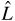.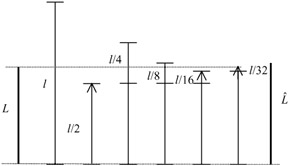Figure 4.11: Principles of successive approximation

The process begins by choosing an initial yardstick length l. The value of l is set to half the largest coefficient in the image. If the coefficient is larger than the yardstick, it is represented with the yardstick value, otherwise its value is set to zero. After each pass the yardstick length is halved and the error magnitude, which is the difference between the original value of the coefficient and its reconstructed value, is compared with the new yardstick. The process is continued, such that the final error is acceptable. Hence, by increasing the number of passes the error in the representation of L bycan be made arbitrarily small.

With regard to Figure 4.11, the quantised length L can be expressed as:

 (4.25)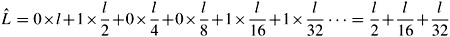where only yardstick lengths smaller than the quantisation error are considered. Therefore, given an initial yardstick l, a length L can be represented as a string of 1 and 0 symbols. As each symbol 1 or 0 is added, the precision in the representation of L increases, and thus the distortion level decreases. This process is in fact equivalent to the binary representation of real numbers, called bit plane representation, where each number is represented by a string of 0s and 1s. By increasing the number of digits, the error in the representation can be made arbitrarily small.

Bit plane quantisation is another form of successive approximation that has been used in some standard codecs such as the JPEG2000 standard. Here, the wavelet coefficients are first represented by their maximum possible precision. This depends on the input pixel resolution (e.g. eight bits) and the dynamic range of the wavelet filter's coefficients. The symbols that represent the quantised coefficients are encoded one bit at a time, starting with the most significant bit (MSB) and preceding to the least significant bit (LSB). Thus for an M-bit plane quantisation with the finest quantiser step size of , the yardstick is 2M-l. ∆ is called the basic quantiser step size.

### Similarities among the bands

A two-stage wavelet transform (seven bands) of the flower garden image sequence with the position of the bands was shown in Figure 4.10. It can be seen that the vertical bands look like scaled versions of each other, as do the horizontal and diagonal bands. Of particular interest in these subimages is the fact that the nonsignificant coefficients from bands of the same orientation tend to be in the same corresponding locations. Also, the edges are approximately at the same corresponding positions. Considering that subimages of lower bands (higher stages of decomposition) have half the dimensions of their higher bands, then one can make a quad tree representation of the bands of the same orientation, as shown in Figure 4.12 for a ten-band (three-stage wavelet transform).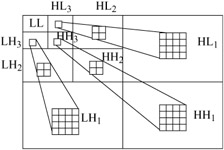Figure 4.12: Quad tree representation of the bands of the same orientation

In this Figure a coefficient in the lowest vertical band, LH3, corresponds to four coefficients of its immediately higher band LH2, which relates to 16 coefficients in LH1. Thus, if a coefficient in LH3 is zero, it is likely that its children in the higher bands of LH2 and LH1 are zero. The same is true for the other horizontal and diagonal bands. This tree of zeros, called zero tree, is an efficient way of representing a large group of zeros of the wavelet coefficients. Here, the root of the zero tree is required to be identified and then the descendant children in the higher bands can be ignored.

## Embedded zero tree wavelet (EZW) algorithm

The combination of the zero tree roots with successive approximation has opened up a very interesting coding tool for not only efficient compression of wavelet coefficients, but also as a means for spatial and SNR scalability .

The encoding algorithm with slight modification on the successive approximation, for efficient coding, according to EZW  is described as follows:

1. The image mean is computed and extracted from the image. This depends on how the lowest band LL is coded. If it is coded independently of other bands, such as with DPCM in MPEG-4, then this stage can be ignored.
2. An R stage (3R+1 band) wavelet transform is applied to the (zero mean) image.
3. The initial yardstick length l is set to half of the maximum absolute value of the wavelet coefficients.
4. A list of the coordinates of the coefficients, called the dominant list, is generated. This list determines the order in which the coefficients are scanned. It must be such that coefficients from a lower frequency band (higher scale) are always scanned before the ones from a higher frequency band. Two empty lists of coefficient coordinates, called the subordinate list and the temporary list, are also created.
5. The wavelet transform of the image is scanned and, if a wavelet coefficient is smaller than the current yardstick length l, it is reconstructed to zero. Otherwise, it is reconstructed as ±3l/2, according to its sign.
6. Dominant pass: the reconstructed coefficients are scanned again, according to the order in the dominant list, generating a string of symbols as follows. If a reconstructed coefficient is positive or negative, a + or a - is added to the string, and the coordinates of this coefficient are appended to the subordinate list. If a reconstructed coefficient is zero, its coordinates are appended to the temporary list. In the case of a zero-valued reconstructed coefficient, two different symbols can be appended to the string; if all its corresponding coefficients in bands of the same orientation and higher frequencies are zero, a zero tree root (ZT) is added to the string, and its corresponding coefficients are removed from the dominant list and added to the temporary list (since they are already known to be zero, they do not need to be scanned again). Otherwise, an isolated zero (Z) is added to the string. The strings generated from the four-symbol alphabet of +, -, ZT and Z are encoded with an adaptive arithmetic encoder , whose model is updated to four symbols at the beginning of this pass. However, during the scanning of the highest horizontal, vertical and diagonal frequency bands (HL1, LH1 and HH1 of Figure 4.12), no zero tree roots can be generated. Therefore, just before the scanning of the first coefficient of these bands, the model of the arithmetic coder is updated to three symbols of +, - and Z.
7. The yardstick length l is halved.
8. Subordinate pass: the coefficients which previously have not been reconstructed as zero are scanned again according to their order in the subordinate list, and each one has added to it either +l/2 or -l/2 in order to minimise the magnitude of its reconstruction error. If l/2 is added, a + is appended to the string, and if l/2 is subtracted, a - is appended. At the end of the subordinate pass the subordinate list is reordered so that the coefficients whose reconstructed values have higher magnitudes come first. The + and - symbols of this pass are encoded with the arithmetic coder, which had its model updated to two symbols (+ and -) at the beginning of this pass.
9. The dominant list is replaced by the temporary list, and the temporary list is emptied.
10. The whole process is repeated from step 5. It stops at any point when the size of the bit stream exceeds the desired bit rate budget.

An observation has to be made on the dominant pass (step 6). In this pass, only the reconstructed values of the coefficients that are still in the dominant list can be affected. Therefore, in order to increase the number of zero tree roots, the coefficients not in the dominant list can be considered zero for determining if a zero-valued coefficient is either a zero tree root or an isolated zero.

The bit stream includes a header giving extra information to the decoder. The header contains the number of wavelet transform stages, the image dimensions, the initial value of the yardstick length and the image mean. Both the encoder and decoder initially have identical dominant lists. As the bit stream is decoded, the decoder updates the reconstructed image, as well as its subordinate and temporary lists. In this way, it can exactly track the stages of the encoder, and can therefore properly decode the bit stream. It is important to observe that the ordering of the subordinate list in step 8 is carried out based only on the reconstructed coefficient values, which are available to the decoder. If it was not so, the decoder would not be able to track the encoder, and thus the bit stream would not be properly decoded.

### Analysis of the algorithm

The above algorithm has many interesting features, which make it especially significant to note. Among them one can say:

1. The use of zero trees exploits similarities among the bands of the same orientation and reduces the number of symbols to be coded.
2. The use of a very small alphabet to represent an image (maximum number of four symbols) makes adaptive arithmetic coding very efficient, because it adapts itself very quickly to any changes in the statistics of the symbols.
3. Since the maximum distortion level of a coefficient at any stage is bounded by the current yardstick length, the average distortion level in each pass is also given by the current yardstick, being the same for all bands.
4. At any given pass, only the coefficients with magnitudes larger than the current yardstick length are encoded nonzero. Therefore, the coefficients with higher magnitudes tend to be encoded before the ones with smaller magnitudes. This implies that the EZW algorithm tends to give priority to the most important information in the encoding process. This is aided by the ordering of the subordinate in step 8. Thus for the given bit rate, the bits are spent where they are needed most.
5. Since the EZW algorithm employs a successive approximation process, the addition of a new symbol (+, -, ZT and Z) to the string just further refines the reconstructed image. Furthermore, while each symbol is being added to the string it is encoded into the bit stream; hence the encoding and decoding can stop at any point, and an image with a level of refinement corresponding to the symbols encoded/decoded so far can be recovered. Therefore, the encoding and decoding of an image can stop when the bit rate budget is exhausted, which makes possible an extremely precise bit rate control. In addition, due to the prioritisation of the more important information mentioned in item d, no matter where in the bit stream the decoding is stopped, the best possible image quality for that bit rate is achieved.
6. Spatial/SNR scalability: in order to achieve spatial or SNR scalability, two different scanning methods are employed in this scheme. For spatial scalability, the wavelet coefficients are scanned in the subband-by-subband fashion, from the lowest to the highest frequency subbands. For SNR scalability, the wavelet coefficients are scanned in each tree from the top to the bottom. The scanning method is defined in the bit stream.

## Set partitioning in hierarchical trees (SPIHT)

The compression efficiency of EZW is to some extent due to the use of arithmetic coding. Said and Pearlman  have introduced a variant of coding of wavelet coefficients by successive approximation, that even without arithmetic coding outperforms EZW (see Figure 4.20). They call it set partitioning in hierarchical trees (SPIHT). Both EZW and SPIHT are spatial tree-based encoding techniques that exploit magnitude correlation across bands of the decomposition. Each generates a fidelity progressive bit stream by encoding, in turn, each bit plane of a quantised dyadic subband decomposition. Both use a significance test on sets of coefficients to efficiently isolate and encode high magnitude coefficients. However, the crucial parts in the SPIHT coding process is the way the subsets of the wavelet coefficients are partitioned and the significant information is conveyed.

One of the main features of this scheme in transmitting the ordering data is that it is based on the fact that the execution path of an algorithm is defined by the results of the comparisons of its branching points. So, if the encoder and decoder have the same sorting algorithm, then the decoder can duplicate the encoder's execution path if it receives the results of the magnitude comparisons. The ordering information can be recovered from the execution path.

The sorting algorithm divides the set of wavelet coefficients, {Ci, j}, into partitioning subsets Tm and performs the magnitude test:

 (4.26)If the decoder receives a no to that answer (the subset is insignificant), then it knows that all coefficients in Tm are insignificant. If the answer is yes (the subset is significant), then a certain rule shared by the encoder and decoder is used to partition Tm into new subset Tm,l and the significant test is then applied to the new subsets. This set division process continues until the magnitude test is done to all single coordinate significant subsets in order to identify each significant coefficient.

To reduce the number of magnitude comparisons (message bits) a set partitioning rule that uses an expected ordering in the hierarchy defined by the subband pyramid is defined (similar to Figure 4.12, used in zero tree coding). In section 4.4.2 we saw how the similarities among the subimages of the same orientation can be exploited to create a spatial orientation tree (SOT). The objective is to create new partitions such that subsets expected to be insignificant contain a huge number of elements and subsets expected to be significant contain only one element.

To make clear the relationship between magnitude comparisons and message bits, the following function is used:

 (4.27)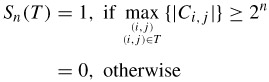to indicate the significance of a set of coordinates T. To simplify the notation of single pixel sets, Sn({(i, j)}) is represented by Sn(i, j).

To see how SPHIT can be implemented, let us assume O(i, j) to represent a set of coordinates of all offsprings of node (i, j). For instance, except for the highest and lowest pyramid levels, O(i, j) is defined in terms of its offsprings as:

 (4.28)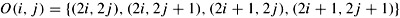We also define D(i, j) as a set of coordinates of all descendants of the node (i, j), and H, a set of coordinates of all spatial orientation tree roots (nodes in the highest pyramid level). Finally, L(i, j) is defined as:

 (4.29)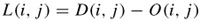The O(i, j), D(i, j) and L(i, j) in a spatial orientation tree are shown in Figure 4.13.Figure 4.13: Spatial orientation tree and the set partitioning in SPIHT

With the use of parts of the spatial orientation trees as the partitioning subsets in the sorting algorithm, the set partitioning rules are defined as follows:

1. The initial partition is formed with the sets {(i, j)} and D(i, j), for all (i, j) ∈ H.
2. If D(i, j) is significant, then it is partitioned into L(i, j) plus the four single-element sets with (k, l) ∈ O(i, j).
3. If L(i, j) is significant, then it is partitioned into the four sets D(k, l), with (k, l) ∈ O(i, j).
4. Each of the four sets now has the format of the original set and the same partitioning can be used recursively.

### Coding algorithm

Since the order in which the subsets are tested for significance is important, in a practical implementation the significance information is stored in three ordered lists, called the list of insignificant sets (LIS), list of insignificant pixels (LIP) and list of significant pixels (LSP). In all lists each entry is identified by a coordinate (i, j), which in the LIP and LSP represents individual pixels, and in the LIS represents either the set D(i, j) or L(i, j). To differentiate between them, it is said that an LIS entry is of type A if it represents D(i, j), and of type B if it represents L(i, j) .

During the sorting pass, the pixels in the LIP, which were insignificant in the previous pass, are tested, and those that become significant are moved to the LSP. Similarly, sets are sequentially evaluated following the LIS order, and when a set is found to be significant it is removed from the list and partitioned. The new subsets with more than one element are added back to the LIS and the single coordinate sets are added to the end of the LIP or the LSP depending whether they are insignificant or significant, respectively. The LSP contains the coordinates of the pixels that are visited in the refinement pass.

Thus the algorithm can be summarised as:

1. Initialisation: let the initial yardstick, n, be n = ⌊log2(max(i, j){|Ci, j|})⌋. Set the LSP as an empty set list, and add the coordinates (i, j) ∈ H to the LIP, and only those with descendants also to the LIS, as the type A entries.
2. Sorting pass:

 2.1 for each entry (i, j) in the LIP do: 2.1.1 output Sn(i, j); 2.1.2 if Sn(i, j) = 1 then move (i, j) to the LSP and output the sign of Ci, j; 2.2 for each entry (i, j) in the LIS do: 2.2.1 if the entry is of type A then 2.2.1.1 output Sn(D(i, j)); 2.2.1.2 if Sn(D(i, j)) = 1 then 2.2.1.2.1 for each (k, l) ∈ O(i, j) do: 2.2.1.2.1.1 output Sn(k, l); 2.2.1.2.1.2 if Sn(k, l) = 1, then add (k, l) to the LSP and output the sign of Ck,l; 2.2.1.2.1.3 if Sn(k, l) = 0 then add (k, l) to the end of the LIP; 2.2.1.2.2 if L(i, j) ≠ Φ then move (i, j) to the end of the LIS, as an entry of type B, and go to step 2.2.2; otherwise, remove entry (i, j) from the LIS; 2.2.2 if the entry is of type B then 2.2.2.1 output Sn(L(i, j)); 2.2.2.2 if Sn(L(i, j)) = 1 then 2.2.2.2.1 add each (k, l) ∈ O((i, j) to the end of the LIS as an entry of type A; 2.2.2.2.2 remove (i, j) from the LIS.
3. Refinement pass: for each entry (i, j) in the LSP, except those included in the last sorting pass (i.e. with the same n), output the nth most significant bit of |Ci,j|.
4. Quantisation step update: decrement n by 1 and go to step 2.

One important characteristic of the algorithm is that the entries added to the end of the LIS above are evaluated before the same sorting pass ends. So, when it is said for each entry in the LIS, it means those that are being added to its end. Also similar to EZW, the rate can be precisely controlled because the transmitted information is formed of single bits. The encoder can estimate the progressive distortion reduction and stop at a desired distortion value.

Note that, in this algorithm, the encoder outputs all branching conditions based on the outcome of the wavelet coefficients. Thus, to obtain the desired decoder's algorithm, which duplicates the encoder's execution path as it sorts the significant coefficients, we simply replace the word output with input. The ordering information is recovered when the coordinate of the significant coefficients is added to the end of the LSP. But note that whenever the decoder inputs data, its three control lists (LIS, LIP and LSP) are identical to the ones used by the encoder at the moment it outputs that data, which means that the decoder indeed recovers the ordering from the execution path.

An additional task done by the decoder is to update the reconstructed image. For the value of n when a coordinate is moved to the LSP, it is known that 2n ≤ |Ci, j| < 2n+1. So, the decoder uses that information, plus the sign bit that is input just after the insertion in the LSP, to set the reconstructed coefficients Ĉi, j = ±1.5 ×2n. Similarly, during the refinement pass, the decoder adds or subtracts 2n-1 to Ĉi, j when it inputs the bits of the binary representation of |Ci,j|. In this manner, the distortion gradually decreases during both the sorting and refinement passes.

Finally it is worth mentioning some of the differences between EZW and SPIHT. The first difference is that they use a slightly different spatial orientation tree (SOT). In EZW, each root node in the top LL band has three offspring, one in each high frequency subband at the same decomposition level and all other coefficients have four children in the lower decomposition subband of the same orientation. However, in SPIHT, in a group of 2 x 2 root nodes in the top LL band, the top left node has no descendant and the other three have four offspring each in the high frequency band of the corresponding orientation. Thus SPIHT uses fewer trees with more elements per tree than in EZW. Another important difference is in their set partitioning rules. SPIHT has an additional partitioning step in which a descendant (type A) set is split into four individual child coefficients and a grand descendant (type B) set. EZW explicitly performs a breadth first search of the hierarchical trees, moving from coarser to finer subbands. Although it is not explicit, SPIHT does a roughly breadth first search as well. After partitioning a grand descendant set, SPIHT places the four new descendant sets at the end of the LIS. It is the appending to the LIS that results in the approximate breadth first traversal.

## Embedded block coding with optimised truncation (EBCOT)

Embedded block coding with optimised truncation EBCOT  is another wavelet-based coding algorithm that has the capability of embedding many advanced features in a single bit stream while exhibiting state-of-the-art compression performance. Due to its rich set of features, modest implementation complexity and excellent compression performance, the EBCOT algorithm has been adopted in the evolving new still image coding standard, under the name of JPEG2000. Thus, because of the important role of EBCOT in the JPEG2000 standard, we describe this coding technique in some detail. Before describing this new method of wavelet coding, let us investigate the problem with EZW and SPIHT that caused their rejection for JPEG2000.

As we will see in Chapter 5, spatial and SNR scalability of images are among the many requirements from the JPEG2000 standard. Spatial scalability means, from the compressed bit stream, to be able to decode pictures of various spatial resolutions. This is in fact an inherent property of the wavelet transform, irrespective of how the wavelet coefficients are coded, since at any level of the decomposition the lowest band of that level gives a smaller replica of the original picture. The SNR scalability means, from the compressed bit stream, to be able to decode pictures of various qualities. In both EZW and SPIHT, this is achieved by successive approximation or bit plane encoding of the wavelet coefficients. Thus it appears that EZW and SPIHT coding of the wavelet coded images can meet these two scalability requirements. However, the bit streams of both EZW and SPIHT inherently offer only SNR scalability. If spatial scalability is required, then the bit stream should be modified accordingly, which is then not SNR scalable. This is because the zero tree-based structure used in these methods involves downward dependencies between the subbands produced by the successive wavelet decompositions. These dependencies interfere with the resolution scalability. Moreover, the interband dependency by the use of zero tree structure causes the error to propagate through the bands. This again does not meet the error resilience requirement of JPEG2000.

These shortfalls can be overcome if each subband is coded independently. Even to make coding more flexible, subband samples can be partitioned into small blocks with each block coded independently. The dependencies may exist within a block but not between different blocks. The size of the blocks determines the degree to which one is prepared to sacrifice coding efficiency in exchange for flexibility in the ordering of information within the final compressed bit stream. The independent block coding paradigm is the heart of EBCOT. This independent coding allows local processing of the samples in each block, which is advantageous for hardware implementation. It also makes highly parallel implementation possible, where multiple blocks are encoded and decoded simultaneously. More importantly, due to flexibility in the rearrangement of bits in the EBCOT, simultaneous SNR and spatial scalability is possible. Obviously, the error encountered in any block's bit stream will clearly have no influence on other blocks and hence improves the robustness. Finally, since unlike EZW and SPHIT similarities between the bands are not exploited, there is small deficiency in the compression performance. This is compensated for by the use of more efficient context-based arithmetic coding and the post compression rate distortion (PCRD) optimisation.

In EBCOT, each subband is partitioned into relatively small block of samples, called code blocks. Typical code block size is either 32 × 32 or 64 × 64 and each code block is coded independently. The actual coding algorithm in EBCOT can be divided into three stages:

1. bit plane quantisation
2. binary arithmetic coding (tier 1 coding)
3. bit stream organisation (tier 2 coding).

Each of these steps is described briefly in the following subsections.

### Bit plane quantisation

All code blocks of a subband use the same quantiser. The basic quantiser step size ∆b of a subband b is selected based on either perceptual importance of the subband or rate control. The quantiser maps the magnitude of a wavelet coefficient to a quantised index, as shown in Figure 4.14, keeping its sign. It has uniform characteristics (equal step size) with a dead zone of twice the step size.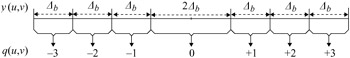Figure 4.14: Uniform dead zone quantiser with step size ∆b

In bit plane coding, the quantiser index is encoded one bit at a time, starting from the most significant bit (MSB) and preceding to the least significant bit (LSB). If K is the sufficient number of bits to represent any quantisation index in a code block, then the maximum coefficient magnitude will be ∆b(2K - 1). An embedded bit stream for each code block is formed by first coding the most significant bit, i.e. (K - 1)th bit together with the sign of any significant sample for all the samples in the code block. Then the next most significant bit, i.e. (K - 2)th bit, is coded until all the bit planes are encoded. If the bit stream is truncated then some or all of the samples in the block may be missing one or more least significant bits. This is equivalent to having used a coarser dead zone quantiser with step size ∆b2P, where p is the index of the last available bit plane for the relevant sample or p least significant bits of quantiser index still remain to be encoded.

### Conditional arithmetic coding of bit planes (tier 1 coding)

During progressive bit plane coding, substantial redundancies exist between the successive bit planes. The EBCOT algorithm has exploited these redundancies in two ways. The first is to identify whether a coefficient should be coded, and the second how best the entropy coding can be adapted to the statistics of the neighbouring coefficients. Both of these goals are achieved through the introduction of the binary significance state σ, which is defined to signify the importance of each coefficient in a code block. At the beginning of the coding process, the significant states of all the samples in the code block are initialised to 0 and then changed to 1 immediately after coding the first nonzero magnitude bit for the sample. Since the neighbouring coefficients generally have similar significance resulting in clusters of similar binary symbols in a plane, then the significance state, σ, is a good indicator for a bit plane of a coefficient to be a candidate for coding.

Also, in EBCOT, adaptive binary arithmetic is used for entropy coding of each symbol. Again, the clustering of significance of neighbours can be exploited to adapt the probability model, based on the significance states of its immediate neighbours. This is called context-based binary arithmetic coding, where the probability assigned to code a binary symbol of 0 or 1 or the positive/negative sign of a coefficient, is derived from the context of its eight immediate neighbours, shown in Figure 4.15.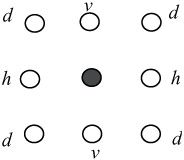Figure 4.15: Eight immediate neighbouring symbols

In general, the eight immediate neighbours can have 256 different contextual states, or as many contextual states for the positive and negative signs. In EBCOT, the probability models used by the arithmetic coder are limited within 18 different contexts: nine for the significance propagation, one for run length, five for sign coding and three for refinement. These contexts are explained in the relevant parts.

Since the status of the eight immediate neighbours affects the formation of the probability model, the way coefficients in a code block are scanned should be defined. In the JPEG2000 standard, in every bit plane of a code block, the coefficients are visited in a stripe scan order with a height of four pixels, as shown in Figure 4.16.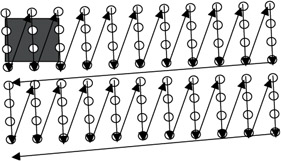Figure 4.16: Stripe scanned order in a code block

Starting from the top left, the first four bits of the first column are scanned. Then the four bits of the second column, until the width of the code block is covered. Then the second four bits of the first column of the next stripe are scanned and so on. The stripe height of four has been chosen to facilitate hardware and software implementations.

### Fractional bit plane coding

The quantised coefficients in a code block are bit plane encoded independent of other code blocks in the subbands. Instead of encoding the entire bit plane in one pass, each bit plane is encoded in three subbit plane passes, called fractional bit plane coding. The reason for this is to be able to truncate the bit stream at the end of each pass to create a near optimum bit stream. This is also known as post compression rate distortion (PCRD) optimisation . Here, the pass that results in the largest reduction in distortion for the smallest increase in bit rate is encoded first.

The goal and benefits of fractional bit plane coding can be understood with the help of Figure 4.17. Suppose (R1, D1) and (R2, D2) are the rate distortion pairs corresponding to two adjacent bit planes p1 and p2. Also, assume during the encoding of each pass that the increase in bit rate and reduction in distortion follow the characteristics identified by labels A, B and C. As we see, in coding the whole bit plane, that is going from point X1 to point X2, no matter which route is followed, the ultimate bit rate is increased from R1 to R2, and the distortion is reduced from D1 to D2. But if due to the limit in the bit rate budget, the bit rate has to be truncated between R1 and R2, R1 ≤ R ≤ R2, then it is better to follow the passes in the sequence ABC rather than CBA.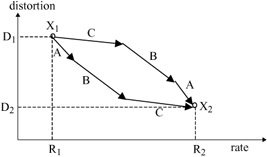Figure 4.17: The impact of order of fractional bit plane coding in distortion reduction

This sort of fractional bit plane coding is in fact a method of optimising the rate distortion curve, with the aim of generating a finely embedded bit stream, which is known as post compression rate distortion (PCRD) optimisation in EBCOT . Figure 4.18 compares the optimised rate distortion associated with the fractional bit plane encoding versus that of the conventional bit plane encoding. The solid dots represent the rate distortion pairs at the end of each biplane, and the solid line is the rate distortion curve that one could expect to obtain by truncating the bit stream produced by this method to an arbitrary bit rate. On the other hand, the end points associated with each pass of the fractional bit plane coding are shown with blank circles and the broken line illustrates its rate distortion curve. Since initial coding passes generally have steeper rate distortion slopes, the end point for each coding pass lies below the convex interpolation of the bit plane termination point. Thus fractional bit plane encoding results in a near optimum coding performance compared with simple bit plane coding.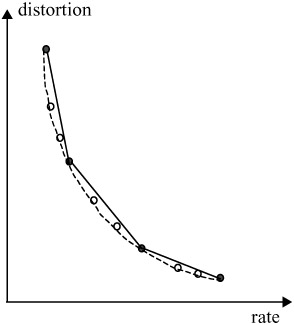Figure 4.18: Rate distortion with optimum trunctaion

Generally, in coding a coefficient the largest reduction in distortion occurs when the coefficient is insignificant, but it is more likely to become significant during the coding. Moderate reduction in distortion is when the coefficient is already significant and the coding refines it. Finally, the least reduction in distortion is when the insignificant coefficient after the encoding is likely to remain insignificant. These are in fact the remaining coefficients not coded in the two previous cases. Thus it is reasonable to divide bit plane encoding into three passes, and encode each pass in the above encoding order. In JPEG2000, the fractional bit plane is carried out in three passes. The roles played by each encoding pass, and their order of significance in generating an optimum bit stream, are given below.

4.7.3.1 Significance propagation pass

This is the first pass of the fractional bit plane encoding that gives the largest reduction in the encoding distortion. In this pass, the bit of a coefficient in a given bit plane is encoded, if and only if, prior to this pass, the state of the coefficient was insignificant but at least one of its eight immediate neighbours had significant states. If the coefficient is to be coded, the magnitude of its bit, 0 or 1, is arithmetically coded with a derived probability model from the context of its eight immediate neighbours, shown in Figure 4.15. The probability assigned to bit 0 is complementary to the probability assigned to bit 1. The context selection is based upon the significance of the sample's eight immediate neighbours, which are grouped in three categories.

 (4.30)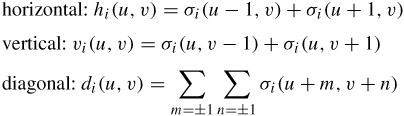where hi(u, v), vi(u, v) and di(u, v) are the horizontal, vertical and diagonal neighbours, for the ith coefficient at coordinates (u, v), and σi (u, v) is the significance state of a coefficient at those coordinates. Neighbours that lie outside the code block are interpreted as insignificant for the purpose of constructing these three quantities. To optimise both the model adaptations of cost and implementation complexity, 256 possible neighbourhood configurations are mapped to nine distinct coding contexts based on eqn. 4.30, as shown in Table 4.4.

Table 4.4: Assignment of the nine contexts based on neighbourhood significance

LL, LH and HL bands

HH band

hi(u, v)

vi(u, v)

di(u, v)

context

di(u, v)

hi(u, v) + vi(u, v)

context

0

0

0

0

0

0

0

0

0

1

1

0

1

1

0

0

>1

2

0

>1

2

0

1

X

3

1

0

3

0

2

X

4

1

1

4

1

0

0

5

1

>1

5

1

0

>0

6

2

0

6

1

>0

X

7

2

>0

7

2

X

X

8

>2

X

8

To make identical context assignment for LH and HL bands, the code blocks of HL subbands are transposed before the coding. The LH subband responds most strongly to horizontal edges in the original image, so the context mapping gives more emphasis on the horizontal neighbours.

Note that the significance propagation pass includes only those bits of coefficients that were insignificant before this pass and have a nonzero context. If the bit of the coefficient is 1 (the coefficient becomes significant for the first time), then its state of significance, σ, is changed to 1, to affect the context of its following neighbours. Thus the significance of states of coefficients propagates throughout the coding, and hence the name given to this pass is the significance propagation pass. Note also that if a sample is located at the boundary of a block, then only the available immediate neighbours are considered and the significance state of the missing neighbours are assumed to be zero.

Finally, if a coefficient is found to be significant, its sign is also arithmetically coded. Since the sign bits from the adjacent samples exhibit substantial statistical dependencies, they can be effectively exploited to improve the arithmetic coding efficiency. For example, the wavelet coefficients of horizontal and vertical edges are likely to be of the same polarity. Those after and before the edge are of mainly opposite polarity. In the EBCOT algorithm, the arithmetic coding of a sign bit employs five contexts. Context design is based upon the relevant sample's immediate horizontal and vertical neighbours, each of which may be in one of the three states: significant and positive, significant and negative, insignificant. There are thus 34 = 81 unique neighbourhood configurations. The details of the symmetry configurations and approximations to map these 81 configurations to one of the five context levels can be found in .

4.7.3.2 Magnitude refinement pass

The magnitude refinement pass is the second most efficient encoding pass. During this pass, the magnitude bit of a coefficient that has already become significant in a previous bit plane is arithmetically coded. The magnitude refinement pass includes the bits from the coefficients that are already significant, except those that have just become significant in the immediately preceding significance propagation pass. There are three contexts for the arithmetic coder, which are derived from the summation of the significance states of the horizontal, vertical and diagonal neighbours. These are the states as currently known to the decoder and not the states used before the significance decoding pass. Further, it is dependent on whether this is the first refinement bit (the bit immediately after the significance and sign bits) or not.

In general, the refinement bits have an even distribution, unless the coefficient has just become significant in the previous bit plane (i.e. the magnitude bit to be encoded is the first refinement bit). This condition is first tested and, if it is satisfied, the magnitude bit is encoded using two contexts, based on the significance of the eight immediate neighbours (see Figure 4.15). Otherwise, it is coded with a single context regardless of the neighbouring values.

4.7.3.3 Clean up pass

All the bits not encoded during the significance propagation and refinement passes are encoded in the clean up pass. That is the coefficients that are insignificant and had the context value of zero (none of the eight immediate neighbours was significant) during the significance propagation pass. Generally, the coefficients coded in this pass have a very small probability of being significant and hence are expected to remain insignificant. Therefore, a special mode, called the run mode, is used to aggregate the coefficients of remaining insignificance. A run mode is entered if all the four samples in a vertical column of the stripe of Figure 4.16 have insignificant neighbours. Specifically, run mode is executed when each of the following conditions holds:

• four consecutive samples must all be insignificant, i.e. σi (u + m, v) = 0, for 0 ≤ m ≤ 3
• the samples must have insignificant neighbours, i.e. hi(u + m, v) = vi (u + m, v) = di(u + m, v) = 0, for 0 ≤ m ≤ 3
• samples must reside within the same subblock
• the horizontal index of the first sample, u, must be even.

In the run mode a binary symbol is arithmetically coded with a single context to specify whether all four samples in the vertical column remain insignificant. Symbol 0 implies that all the four samples are insignificant and symbol 1 implies that at least one of four samples becomes significant in the current bit plane. If the symbol is 1, then two additional arithmetically coded bits are used to specify the location of the first nonzero coefficient in the vertical column.

Since it is equally likely that any one of the four samples in the column is the first nonzero sample, then the arithmetic coding uses a uniform context. Thus run mode has a negligible role in coding efficiency. It is primarily used to improve the throughput of the arithmetic encoder through symbol aggregation.

After specifying the position of the first nonzero symbol in the run, the remaining samples in the vertical column are coded in the same manner as in the significance propagation pass and use the same nine contexts. Similarly, if at least one of the four coefficients in the vertical column has a significant neighbour, the run mode is disabled and all the coefficients in that column are again coded with the procedure used for the significance propagation pass.

For each code block, the number of MSB planes that are entirely zero is signalled in the bit stream. Since the significance state of all the coefficients in the first nonzero MSB is zero, this plane only uses the clean up pass and the other two passes are not used.

Example

In order to show how the wavelet coefficients in a fractional bit plane are coded, Figure 4.19 illustrates a graphical demonstration of step-by-step encoding from bit plane to bit plane and pass to pass.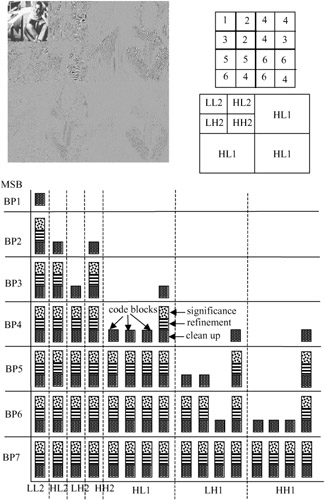Figure 4.19: An illustration of fractional bit plane encoding

The Barbara image of size 256 x 256 pixels with two levels of wavelet decomposition generates seven subimages, as shown in Figure 4.19. Except for the lowest band, the magnitudes of all the other bands are magnified by a factor of four, for better illustration of image details. The code block size is assumed to be a square array of 64 x 64 coefficients. Hence, every high frequency band of LH1, HL1 and HH1 is coded in four code blocks, and the remaining bands of LL2, LH2, HL2 and HH2 in one code block each. That is, the whole image is coded in 16 code blocks. The bit streams generated by each pass in every bit plane are also shown in square boxes with different textures.

Before the coding starts, the significance states of all the code blocks are initialised to zero. For each code block the encoding starts from the most significant bit plane. Since the LL2 band has a higher energy (larger wavelet coefficients) than the other bands, in this example only some of the MSB of the code block of this band are significant at the first scanned bit plane, PB1. In this bit plane the MSB of none of the other code blocks is significant (they are entirely zero), and are not coded at all.

Since the code block of LL2 band in BP1 is coded for the first time (the significant states of all the coefficients are initialised to zero), then the MSB bit of every coefficient has a nonsignificant neighbour and hence cannot be coded at the significance propagation pass. Also, none of the bits are coded at the refinement pass, because these coefficients had not been coded in the previous bit plane. Therefore, all the bits are left to be coded in the clean up pass, and they constitute the bit stream of this pass.

At the second bit plane, BP2, some of the coefficients in the HL2 and HH2 code blocks become significant for the first time. Hence, as was explained earlier, they are coded only at the clean up pass, as shown in the Figure. For the code block of LL2, since the coefficients with significant magnitudes of this code block have already been coded at the clean up pass of BP1, the block uses all the three passes. Those coefficients of the bit plane BP2 with insignificant states that have at least one state significant immediate neighbour are coded with the significance propagation pass. The state significant coefficients are now refined in the refinement pass. The remaining coefficients are coded at the clean up pass. Other code blocks in this bit plane are not coded at all.

At the third bit plane, BP3, some of the coefficients of the code block of subband LH2 and those of the code block of subband HL1 become significant for the first time, hence they are coded only in the clean up pass. The code blocks of LL2, HL2 and HH2 are now coded at the three passes. The remaining code blocks are not coded at all. The bit plane numbers of those code blocks that are coded for the first time are also shown in the Figure with numbers from 1 to 6. As we see, after bit plane 7 all the code blocks of the bands are coded in all three passes.

### Layer formation and bit stream organisation (tier 2 coding)

The arithmetic coding of the bit plane data is referred as tier 1 coding. The tier 1 coding generates a collection of bit streams with one independent embedded bit stream for each code block. The purpose of tier 2 coding is to multiplex the bit streams for transmission and to signal the ordering of the resulting coded bit plane pass in an efficient manner. The second tier coding process can be best viewed as a somewhat elaborated parser for recovering pointers to code block segments in the bit stream. It is this coding step which enables the bit stream to have SNR, spatial and arbitrary progression and scalability.

The compressed bit stream from each code block is distributed across one or more layers in the final compressed bit stream. Each layer represents a quality increment. The number of passes included in a specific layer may vary from one code block to another and is typically determined by the encoder as a result of PCRD optimisation. The quality layers provide the feature of SNR scalability of the final bit stream such that truncating the bit stream to any whole number of layers yields approximately an optimal rate distortion representation of the image. However, employing a large number of quality layers can minimise the approximation. On the other hand, more quality layers implies a large overhead as auxiliary information to identify the contribution made by each code block to each layer. When the number of layers is large, only a subset of the code blocks will contribute to any given layer, introducing substantial redundancy in this auxiliary information. This redundancy is exploited in tier 2 coding to efficiently code the auxiliary information for each quality layer.

### Rate control

Rate control refers to the process of generating an optimal image for a bit rate and is strictly an encoder issue. In section 4.7.3 we introduced fractional bit plane coding as one of these methods. In , Taubman proposes an efficient rate control method for the EBCOT compression algorithm that achieves a desired rate in a single iteration with minimum distortion. This method is known as post compression rate distortion (PCRD) optimisation. A JPEG2000 encoder with several possible variations can also use this method.

In another form of PCRD, each subband is first quantised using a very fine step size, and the bit planes of the resulting code blocks are entropy coded (tier 1 coding). This typically generates more coding passes for each code block than will be eventually included in the final bit stream. Next, a Lagrangian R-D optimisation is performed to determine the number of coding passes from each code block that should be included in the final compressed bit stream to achieve the desired bit rate. If more than a single quality layer is desired, this process can be repeated at the end of each layer to determine the additional number of coding passes from each code block that need to be included in the next layer. The details of PCRD optimisation can be found in .

At the end of this Chapter, it is worth comparing the compression efficiency of the three methods of wavelet coding, namely: EZW, SPIHT and the EBCOT. Figure 4.20 shows the quality of the Lena image coded with these methods at various bit rates. As the Figure shows, EZW has the poorest performance of all. The SPIHT method, even without arithmetic coding, outperforms EZW by about 0.3–0.4 dB. Adding arithmetic coding into SPIHT improves the coding efficiency by another 0.3 dB. The EBCOT algorithm, adopted in the JPEG2000 standard, is as good as the best of SPIHT.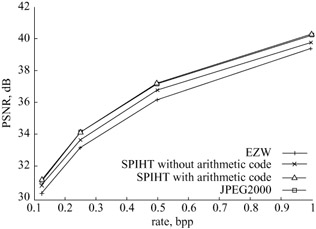Figure 4.20: Compression performance of various wavelet coding algorithms

## Problems

 1 Derive the analysis and synthesis subband filters of the product filter P(z) defined by its z transform as:2 The z transform of a pair of lowpass and highpass analysis filters is given by: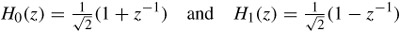Calculate the product filter, and deduce the amount of end to end encoding/decoding delay Derive the corresponding pairs of the synthesis filters.3 The product filter for wavelets with n zeros at z = -1 is given by: P(z) = (1 + z-1)2nQ(z) Use eqn. 4.7 to calculate the weighting factor of the product filter P(z) and the corresponding end-to-end coding delay, for: n = 2 and Q(z) = -1 + 4z-1 - z-2 n = 3 and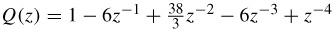4 Show that the low and highpass analysis filters of a in problem 3 are in fact the (5, 3) and (4, 4) subband filters of LeGall and Tabatabai.5 Show that the filters for b in problem 3 are the 9/3 Daubechies filter pairs.6 Derive the corresponding pairs of the synthesis filters of problems 4 and 5.7 List major similarities and differences between EZW and SPIHT.8 Consider a simple wavelet transform of 8 x 8 image shown in Figure 4.21. Assume it has been generated by a three-level wavelet transform: Show different steps in the first dominant pass (most significant bit plane) of the EZW algorithm Assuming that EZW uses two bits to code symbols in the alphabet {POS, NEG, ZT, Z} and one bit to code the sign bit, calculate total number of bits outputted in this pass.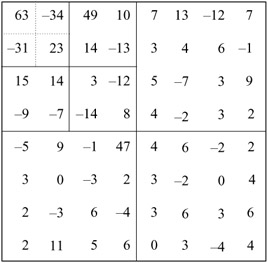Figure 4.219 In Figure 4.21, calculate the number of bits outputted in the first significant pass of the SPIHT algorithm Comment on the results of 8b and 9a.Answers

 1 P(z) = H0 (z)H1 (-z) should be factorised into two terms. The given P(z) is zero at z-1 = -1, hence it is divisible by 1 + z-1. Divide as many times as possible, that gives: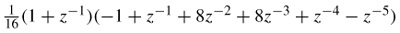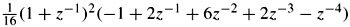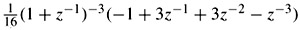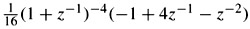Thus in (i) the lowpass analysis filter will be: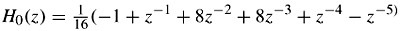and the high pass analysis filter is: H1(z) = 1 - z-1 In (ii)and the highpass: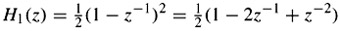which are the (5,3) subband filter pairs. In (iii)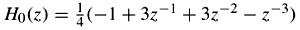and the highpass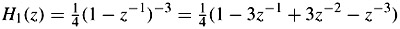which gives the second set of (4,4) subband filters. In (iv)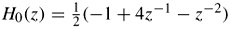and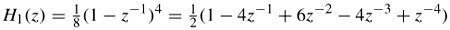Any other combinations may be used, as desired. 2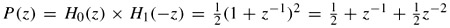. Thus with P(z) - P(-z) = 2z-1, results in one sample delay.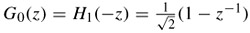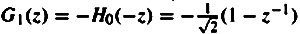3 With a weighting factor of k, P(z) = k(1 + z-1)-4(-l + 4z-1-z-2), and using P(z) - P(-z) = 2z-m, gives k = 1/16 and m = 3. The factor for the other set will be k = 3/256 and m = 5 samples delay. 4 In problem 3, P(z) is in fact type (iv) of problem 1. Hence it leads not only to the two sets of (5,3) and (4,4) filter pairs, but also to two new types of filter, given in (i) and (iv) of problem 1. 5 With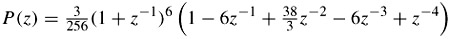retaining H1(-z) = (1 + z-1)2 = 1 + 2z-1 + z-2, that gives the three-tap highpass filter of H1(z) = 1 - 2z-1 + z-2 and the remaining parts give the nine-tap lowpass filter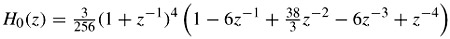or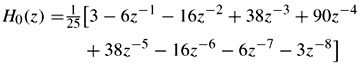Had we divided the highpass filter coefficients, H1(z), by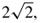, and hence multiplying those of lowpass H0(z) by this amount, we get the 9/3 tap filter coefficients of Table 4.2. 6 Use G0(z) = H1(-z) and G1(z) = -H0(-z) to derive the synthesis filters 7 See pages 84 and 88 8 33 bits 9 29 bits

## References

1 MPEG-4: 'Video shape coding'. ISO/IEC JTC1/SC29/WG11, N1584, March 1997

2 SKODRAS, A.,CHRISTOPOULOS, C., and EBRAHIMI, T.: 'The JPEG 2000 still image compression standard', IEEE Signal Process. Mag., September 2001, pp.36–58

3 MALAVER, H.S., and STAELIN, D.H.: 'The LOT: transform coding without blocking effects', IEEE Trans. Acoustic, Speech Signal Process., 1989, 37:4, pp.553–559

4 SHAPIRO, J.M.: 'Embedded image coding using zero-trees of wavelet coefficients', IEEE Trans. Signal Process., 1993, 4:12, pp.3445–3462

5 USEVITCH, B.E.: 'A tutorial on modern lossy wavelet image compression: foundation of JPEG 2000', IEEE Signal Process. Mag., September 2001, pp.22–35

6 CROCHIERE, R.E.,WEBER, S.A., and FLANAGAN, J.L.: 'Digital coding of speech in sub-bands', Bell Syst. Tech. J., 1967, 55, pp.1069–1085

7 DAUBECHIES, I.: 'Orthonormal bases of compactly supported wavelets', Communications on Pure and Applied Mathematics, 1988, XLI, pp.909–996

8 LE GALL, D., and TABATABAI, A.: 'Subband coding of images using symmetric short kernel filters and arithmetic coding techniques'. IEEE international conference on Acoustics, speech and signal processing, ICASSP'98, 1988, pp.761–764

9 DAUBECHIES, I.: 'The wavelet transform, time frequency localization and signal analysis', IEEE Trans. Inf. Theory, 1990, 36:5, pp.961–1005

10 MALLAT, S.: 'A theory of multi-resolution signal decomposition: the wavelet representation', IEEE Trans. Pattern Anal. Mach. Intell., 1989, 11:7, pp.674–693

11 DAUBECHIES, I.: 'Orthogonal bases of compactly supported wavelets II, variations on a theme', SIAM J. Math. Anal., 1993, 24:2, pp.499–519

12 DA SILVA, E.A.B.: 'Wavelet transforms in image coding'. PhD thesis, 1995, University of Essex, UK

13 RABBANI, M., and Josh, R.: 'An overview of the JPEG2000 still image compression standard', Signal Process., Image Commun., 2002, 17:1, pp.15–46

14 MPEG-4: 'Video verification model version-11'. ISO/IEC JTC1/SC29/WG11, N2171, Tokyo, March 1998

15 WITTEN, I.H.,NEAL, R.M., and CLEARY, J.G.: 'Arithmetic coding for data compression', Commun. ACM, 1987, 30:6, pp.520–540

16 SAID, A., and PEARLMAN, W.A.: 'A new, fast and efficient image codec based on set partitioning in hierarchical trees', IEEE Trans. Circuits Syst. Video Technol., 1996, 6:3, pp.243–250

17 TAUBMAN, D.: 'High performance scalable image compression with EBCOT', IEEE Trans. Image Process., 2000, 9:7, pp.1158–1170

18 TAUBMAN, D.,Ordenlich, E.,WEINBERGER, M., and SEROUSSI, G.: 'Embedded block coding in JPEG2000', Signal Process., Image Commun., 2002, 17:1, pp. 1–24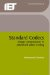Standard Codecs: Image Compression to Advanced Video Coding (IET Telecommunications Series)
ISBN: 0852967101
EAN: 2147483647
Year: 2005
Pages: 148
Authors: M. Ghanbari

Similar book on Amazon

Flylib.com © 2008-2017.
If you may any questions please contact us: flylib@qtcs.net